# Single Cell Generative Pre-trained Transformer

## Results

### Integration of Multiple scRNA-seq data with batch correction

Clustering and visualization of single-cell sequencing data encounter a significant challenge in the presence of batch effects arising from the utilization of multiple datasets or sequencing batches as input.

benchmark scGPT with three popular integration methods

• scVI
• Seurat
• Harmony

on two integration datasets

• Immune Human (10 batches)
• PBMC 10K (2 batches)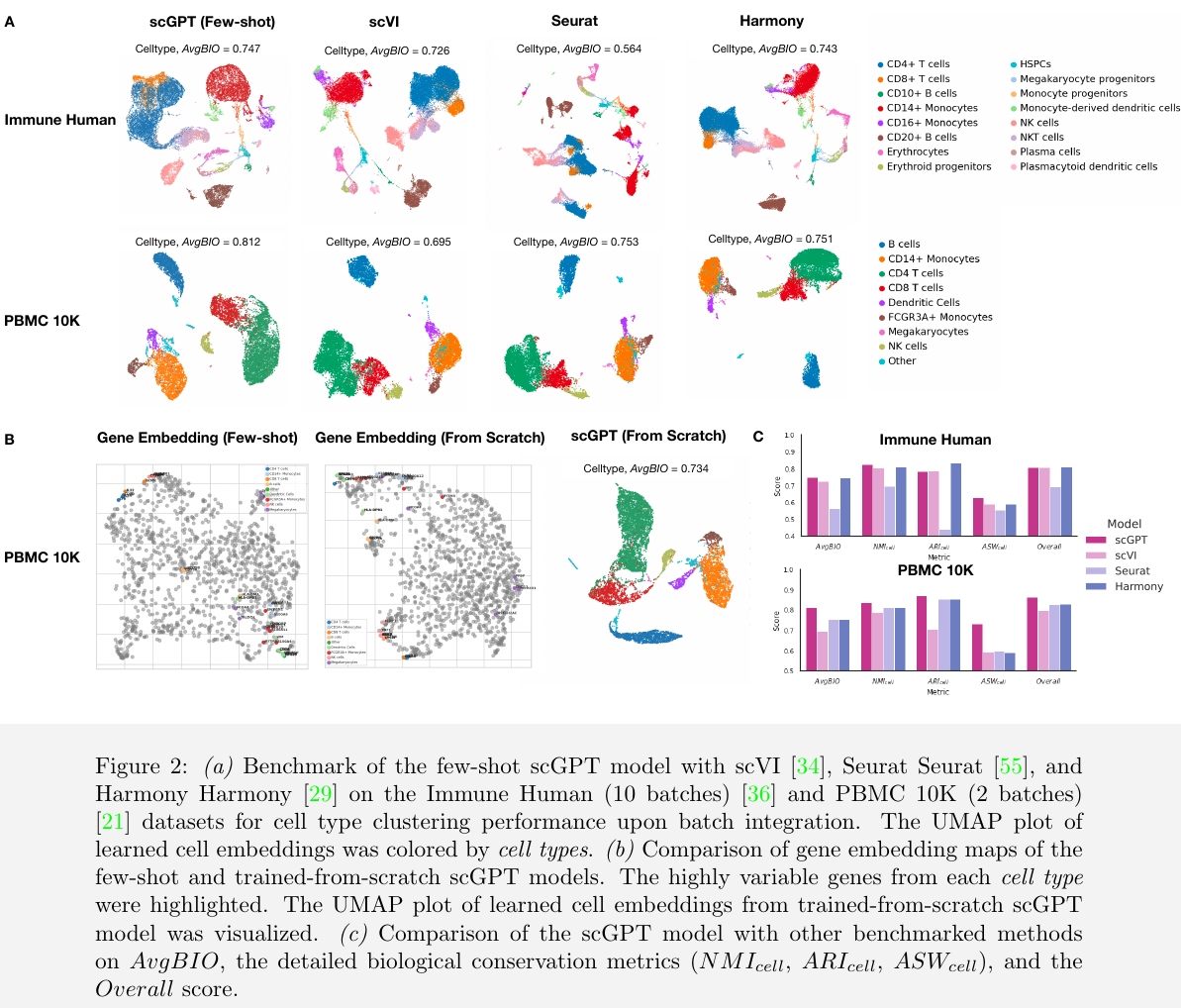### Cell type annotation

Cell type annotation is a crucial step in single-cell analysis after clustering, as it resolves heterogeneity in sequenced tissues and lays the foundation for further investigation of cell and gene functions to gain biological and pathological insights.

several methods have been proposed for cell annotation

• cellAlign
• singleR
• Chetah

they typically require dimension reduction prior to model input, which can lead to information loss.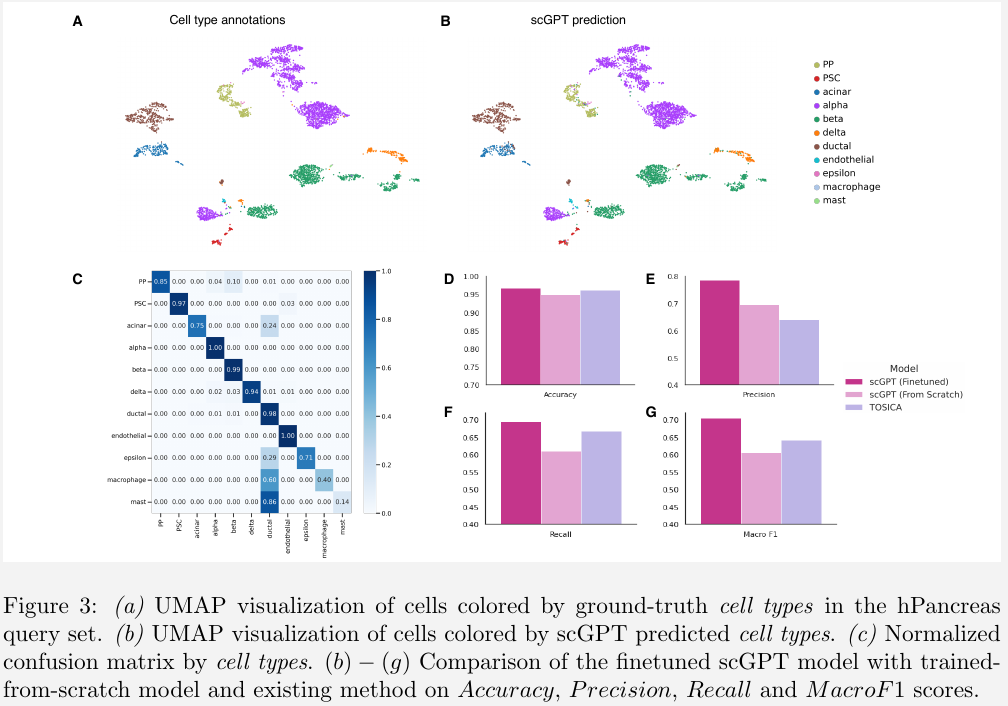finetune the pre-trained scGPT model using cross-entropy loss against ground-truth labels from a new reference dataset.

using the hPancreas dataset of human pancreas cells as an example, train the scGPT model on the reference set and validated the classification performance on a different query set.

### Perturbation Prediction

evaluate the model using two perturbation datasets

• the Pertub-seq dataset of K562 leukemia cell line, which comprises 87 one-gene perturbations, with approximately 100 cells per perturbation and a minimum of 7000 unperturbed cells
• the other Norman Perturb-Seq dataset, consisting of 131 two-gene perturbations and 105 one-gene perturbations

assess the perturbation prediction by calculating the Pearson correlation between the predicted and the corresponding ground-truth expression values after perturbation.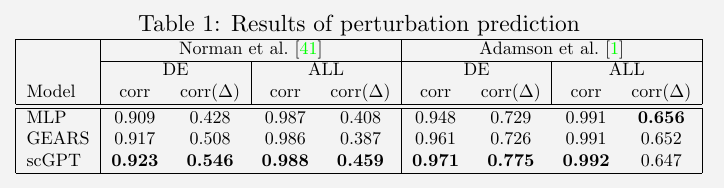### Multi-omic integration and multi-modal representation learning

Single-cell multi-omic (scMultiomic) data presents multiple views of genetic regulation all at once, including epigenetic, transcriptomic, and translation activities.

The challenge is how to reliably aggregate cell representations from multiple views while preserving biological views.

use the 10X Multiome PBMC dataset with joint gene expression and chromatin accessibility measurements

benchmark scGPT with two state-of-the-art methods

• scGLUE
• Seurat v4

on cell type clustering performance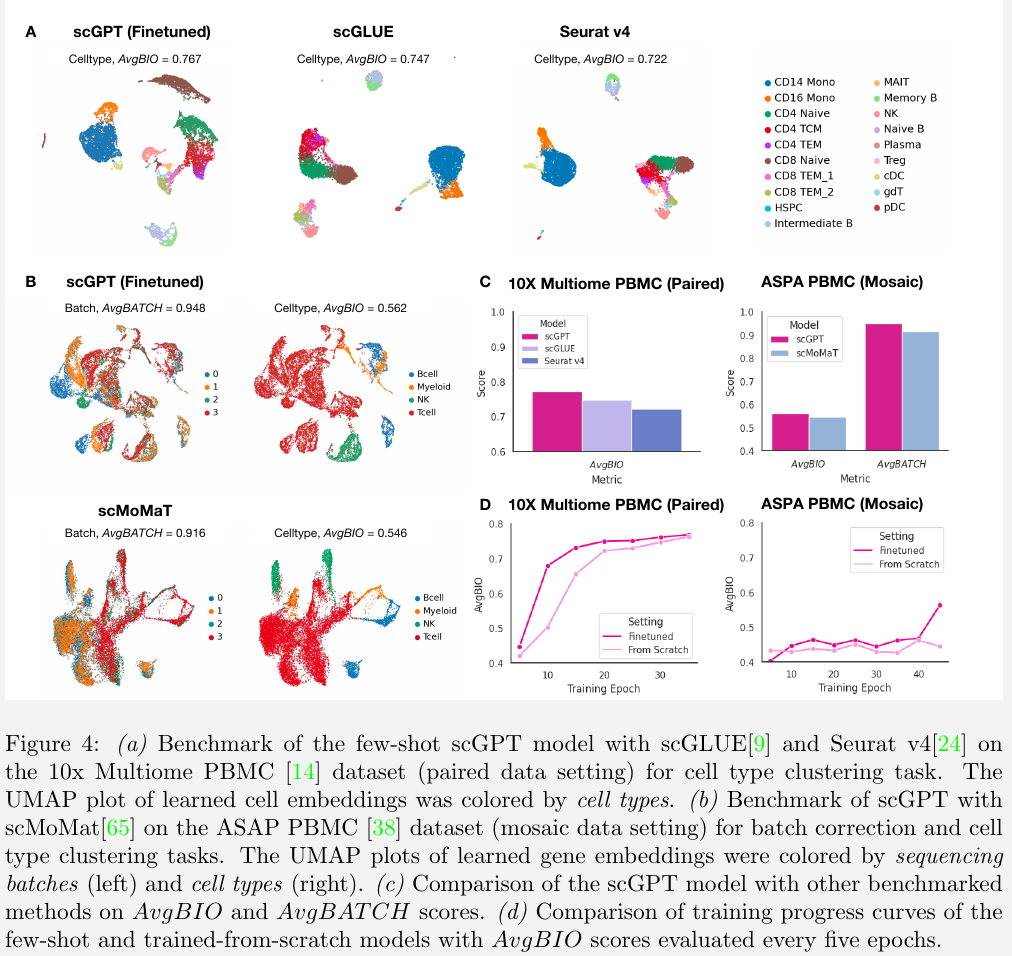### Gene embeddings for Gene Regulatory Network Inference

The interactivity between transcription factors, cofactors and target genes underlying a Gene Regulatory Network (GRN) mediates important biological processes.

Existing GRN inference methods often rely on correlation in static gene expressions or pseudo-time estimates as a proxy for causal graphs.

scGPT demonstrates its ability to group the functionally related genes and differentiate functionally different genes from its gene embedding network

In the zero-shot setting, the scGPT model highlights two clusters corresponding to the two well-characterized HLA classes that trigger different immune responses

• HLA class I antigens HLA-A, -C and -E are recognized by CD8+ T cells to mediate cell killing.
• HLA class II antigens HLA-DR, -DP and -DQ are recognized by CD4+T cells to trigger broader helper functions

scGPT reconstructs meaningful gene programs in a purely unsupervised workflow.

Figure 5D:

• the same HLA antigen cluster was identified as group 1
• the CD3 genes involved in T3 complex were identified as group 4, with highest expressions in T cells

validate the gene similarity relationships encoded by the scGPT model against the known Reactome database.## Methods

### Input embeddings

the single-cell sequencing data is processed into a cell-gene matrix, $X\in \IR^{N\times G}$, where each element $X_{i,j}\in\IR^+$ represents the read count of a RNA for scRNA-seq or a peak region if scATAC-seq

the input to scGPT consists of three main components:

• gene (or peak) tokens
• expression values
• condition tokens

For each modeling task, the gene tokens and expression values are pre-processed from the raw count matrix $X$

• gene tokens: use gene names as tokens, and assign each gene $g_j$ a unique integer identifier $id(g_j)$ within the complete vocabulary of tokens
• incorporate special tokens in the vocabulary, such as <cls> for aggregating all genes into a cell representation
• <pad> for padding the input to a fixed length

the input gene tokens of each cell $i$ are represented by a vector $t_g^{(i)}\in \bbN^M$

$t_g^{(i)} = [id(g_1^{(i)}), id(g_2^{(i)}),\ldots, id(g_M^{(i)})]$

where $M$ is a pre-defined input length, and usually equals to the number of selected highly variable genes.

#### Expression values

value binning techniques

#### Condition tokens

encompass diverse meta information associated with individual genes, such as functional pathways (represented by pathway tokens) or perturbation experiment alterations (indicated by perturbation tokens)

use an input vector that shares the same dimension as the input genes

$t_c^{(i)} = [t_{c, 1}^{(i)}, t_{c, 2}^{(i)},\ldots, t_{c,M}^{(i)}]$

where $t_{c,j}^{(i)}$ represents an integer index corresponding to a condition.

#### Embedding layers

use the conventional embedding layers $emb_g$ and $emb_c$ for the gene tokens and condition tokens, respectively

use fully connected layers, denoted as $emd_x$, for the binned expression values to enhance expressivity.

the final embedding $h^{(i)}\in \IR^{M\times D}$ for cell $i$ is defined as

$h^{(i)} = emb_g(t_g^{(i)}) + emb_x(x^{(i)}) + emb_c(t_c^{(i)})$

## Cell and gene expression modeling by transformers

### scGPT Transformer

the self-attention mechanism operates on the sequence of $M$ embedding vectors

The output of the stacked transformer blocks can be defined as follows

$h_0^{(i)} = h^{(i)}\\ h_l^{(i)} = \text{transformer_block}(h_{l-1}^{(i)}) \forall l\in [1, n]$

use the resulting representation $h_n^{(i)}\in \IR^{M, D}$ for both gene-level and cell-level tasks.

### Cell representation

the representation $h_c^{(i)}\in \IR^D$ is obtained by aggregating the learned gene-level representations $h_n^{(i)}$.

### Condition tokens for batch and modality

• modality tokens $t_m^{(i)}$ are associated with individual input features $g_j$ (to indicate whether it is a gene, region or protein)
• the batch tokens are on the cell level originally but can be propagated to all features of a single cell as well. In other words, the same batch token $t_b^{(i)}$ can be repeated up to the length $M$ of input features of single cell $i$
$t_b^{(i)} = [t_{b,1}^{(i)},\ldots, t_{b,M}^{(i)}] = [t_b^{(i)},\ldots, t_b^{(i)}]$

the batch and modality tokens are not used as input to the transformer blocks

in the scMultiomic integration task, concatenate the transformer output with the sum of batch and modality embeddings. This serves as input to the downstream find-tuning objectives for expression modelling

$h_n'{}^{(i)} = concat(h_n^{(i)}, emb_b(t_b^{(i)}) + emd_m(t_m^{(i)}))$

## Generative pre-training

### Foundation model pre-training

restrict the input to only genes with non-zero expressions for each input cell

### Attention mask for generative pre-training

self-attention has been widely used to capture the co-occurrence patterns among tokens

In NLP, two ways

• masked token prediction used in transformer encoder models such as BERT and Roberta, where randomly masked tokens in the input sequence are predicted in the model’s output
• auto-regressive generation with sequential prediction in causal transformer decoder models such as the OpenAI GPT series

• generating unknown gene expression values based on known gene expressions, i.e., generation by “gene prompts”
• generating whole genome expressions given an input cell type condition, i.e., generation by “cell prompts”

challenge: non-sequential nature of the data

For an input $h_l^{(i)}\in \IR^{M\times D}$ of $M$ tokens, the transformer block will generate $M$ query and key vectors to compute the attention map $A\in \IR^{M\times M}$. The attention mask is of the same size $M\times M$.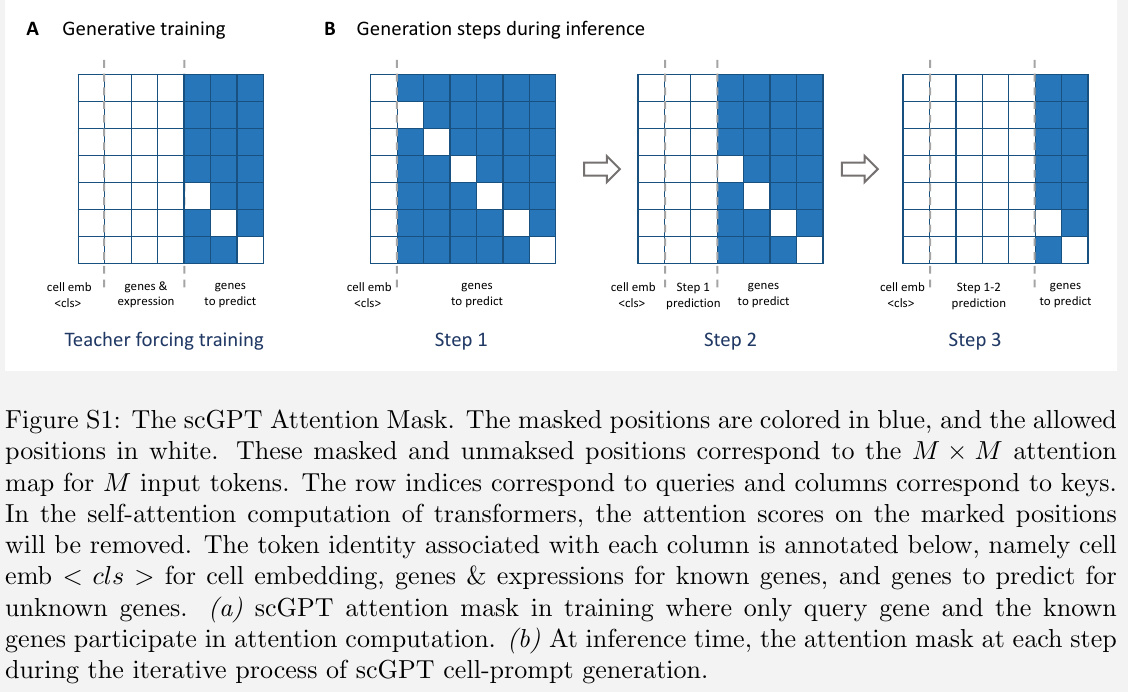The queries on the positions of these unknown genes are only allowed with attention computation on the known genes and the query gene itself.

### Finetuning Objectives

#### Gene Expression Prediction (GEP)

within each cell, a subset of genes and their corresponding expression values $x^{(i)}$ are randomly masked, scGPT is optimized to accurately predict the expression values at the masked positions.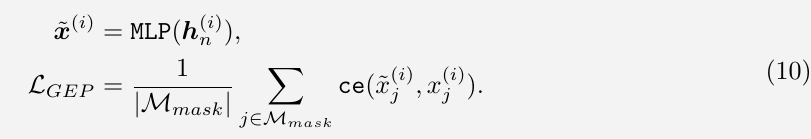#### Gene Expression Prediction for Cell Modelling (GEPC)

similar to GEP, but predict gene expression values based on the cell representation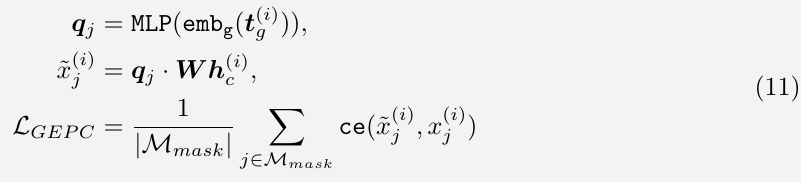#### Elastic Cell Similarity (ECS)

enhance the similarity between pairs exhibiting cosine similarity values above $\beta$

#### Domain Adaptation via Reverse Back-propagation (DAR)

use a distinct MLP classifier to predict the sequencing batch associated with each input cell.

#### Cell Type Classification (CLS)

use a separate MLP classifier to predict the cell types from their cell representations $h_c^{(i)}$